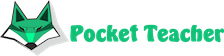# 5 equations that the entire internet is puzzling over

Many scientists recommend training your brain by solving mathematical equations. Thanks to modern technologies, on the Internet you can find a large number of different puzzles that help you develop your abilities. The more you exercise, the faster you can calculate algebra in the future.

## Examples and ways to solve them

The five most popular puzzles are:

• An example in two steps without parentheses. Netizens argue over the result of the problem: 230-220 * 0.5. Someone thinks that the answer is 120, while others are sure that the result is 5. In fact both are right. Only in the second case, the result is 5 with an exclamation mark, that is five with a factorial. 5! = 5 * 4 * 3 * 2 * 1 = 120.
• Equation with brackets. Another subject of controversy on the Internet is the example: 8/2 * (2 + 2). Spread in significant answers: some believe that the result will be 1, while others - 16. If you follow the rules of mathematics, then first the action in brackets is performed, that is, 2 + 2 = 4, and then everything is performed in order. This means 8/2 * 4 = 16. If the author of the example would put one more parentheses before 2, then 2 would be multiplied by 4, and then 8 would be divisible by 8, and in the end the answer would be 1.
• Mindfulness puzzle. In front of users is a picture showing tracks for racing cars, each of which has numbers: 16, 06, 68, 88, one more is closed by a car, and the last one is 98. You are asked to calculate what number is hidden under the car. To solve the problem, you do not need to apply math skills. It is enough just to turn the image over and understand that it is upside down 16 is 91, 06-90, and so on. So the number under the car is 87.
• The principle of constructing examples. Problems of this kind are often encountered. For example, 6 + 4 = 210 or 8 + 5 = 313. The solution is pretty easy. The authors simply first subtracted the number 4 from 6, and then added. It turned out 6-4 = 2 and 6 + 4 = 10, and together 210. Other similar examples are constructed in the same way.
• Equations with pictures. On the Internet you can find many pictures, where, for example, multiply first 3 ice cream cones and the answer is 27, then two full ice creams are added with white vanilla, which is 10 and other actions. You are asked to determine the numbers that inserted by the author instead of pictures. To determine, you must first solve the first example and then substitute this figure in the rest to solve the whole problem.

If you don’t have time to think long and you don’t know, how to solve math , you can do it using our website.Our artificial intelligence solves complex math problems in seconds.

We will solve your exam, homework, olympiad problems with detailed steps. You will need just to copy the solution.